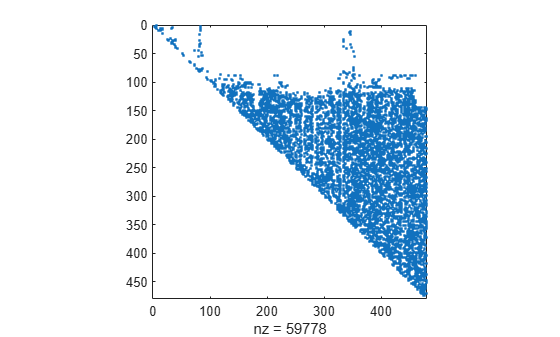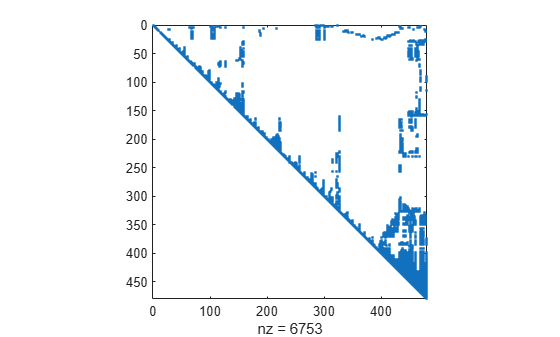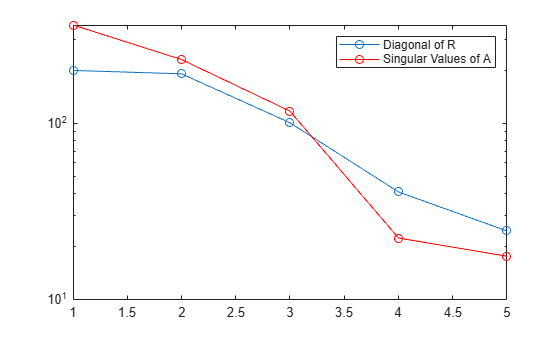qr

QR decomposition

Description

example

X = qr(A) returns the upper-triangular R factor of the QR decomposition A = Q*R. If A is full, then R = triu(X). If A is sparse, then R = X.

example

[Q,R] = qr(A) performs a QR decomposition on m-by-n matrix A such that A = Q*R. The factor R is an m-by-n upper-triangular matrix, and the factor Q is an m-by-m orthogonal matrix.

example

[Q,R,P] = qr(A) additionally returns a permutation matrix P such that A*P = Q*R.

example

[___] = qr(A,0) produces an economy-size decomposition using any of the previous output argument combinations. The size of the outputs depends on the size of m-by-n matrix A:

• If m > n, then qr computes only the first n columns of Q and the first n rows of R.

• If m <= n, then the economy-size decomposition is the same as the regular decomposition.

• If you specify a third output with the economy-size decomposition, then it is returned as a permutation vector such that A(:,P) = Q*R.

example

[Q,R,P] = qr(A,outputForm) specifies whether to return the permutation information P as a matrix or a vector. For example, if outputForm is 'vector', then A(:,P) = Q*R. The default value of outputForm is 'matrix' such that A*P = Q*R.

example

[C,R] = qr(S,B) computes C = Q'*B and the upper-triangular factor R. You can use C and R to compute a least-squares solution to the sparse linear system S*X = B with X = R\C.

example

[C,R,P] = qr(S,B) additionally returns a permutation matrix P. You can use C, R, and P to compute a least-squares solution to the sparse linear system S*X = B with X = P*(R\C).

example

[___] = qr(S,B,0) produces an economy-size decomposition using any of the previous output argument combinations. The size of the outputs depends on the size of m-by-n sparse matrix S:

• If m > n, then qr computes only the first n rows of C and R.

• If m <= n, then the economy-size decomposition is the same as the regular decomposition.

• If you specify a third output with the economy-size decomposition, then it is returned as a permutation vector such that the least-squares solution to S*X = B is X(P,:) = R\C.

example

[C,R,P] = qr(S,B,outputForm) specifies whether to return the permutation information P as a matrix or vector. For example, if outputForm is 'vector', then the least-squares solution to S*X = B is X(P,:) = R\C. The default value of outputForm is 'matrix' such that the least-squares solution to S*X = B is X = P*(R\C).

Examples

collapse all

Find the QR decomposition of the 5-by-5 Pascal matrix. Specify one output argument to return just the upper-triangular factor.

A = pascal(5);
X = qr(A)
X = 5×5

-2.2361   -6.7082  -15.6525  -31.3050  -56.3489
0.3090    3.1623   11.0680   26.5631   53.1263
0.3090   -0.1744    1.8708    7.4833   19.2428
0.3090   -0.4565    0.3548    0.6325    2.8460
0.3090   -0.7387   -0.0281   -0.7490   -0.1195

Extract the upper-triangular factor R from X.

R = triu(X)
R = 5×5

-2.2361   -6.7082  -15.6525  -31.3050  -56.3489
0    3.1623   11.0680   26.5631   53.1263
0         0    1.8708    7.4833   19.2428
0         0         0    0.6325    2.8460
0         0         0         0   -0.1195

Compare the R in the Q-less QR decomposition to the R factor in a full QR decomposition.

[Q1,R1] = qr(A)
Q1 = 5×5

-0.4472   -0.6325    0.5345   -0.3162   -0.1195
-0.4472   -0.3162   -0.2673    0.6325    0.4781
-0.4472    0.0000   -0.5345   -0.0000   -0.7171
-0.4472    0.3162   -0.2673   -0.6325    0.4781
-0.4472    0.6325    0.5345    0.3162   -0.1195

R1 = 5×5

-2.2361   -6.7082  -15.6525  -31.3050  -56.3489
0    3.1623   11.0680   26.5631   53.1263
0         0    1.8708    7.4833   19.2428
0         0         0    0.6325    2.8460
0         0         0         0   -0.1195

Compute the full QR decomposition of a magic square test matrix by specifying two output arguments.

A = magic(5);
[Q,R] = qr(A)
Q = 5×5

-0.5234    0.5058    0.6735   -0.1215   -0.0441
-0.7081   -0.6966   -0.0177    0.0815   -0.0800
-0.1231    0.1367   -0.3558   -0.6307   -0.6646
-0.3079    0.1911   -0.4122   -0.4247    0.7200
-0.3387    0.4514   -0.4996    0.6328   -0.1774

R = 5×5

-32.4808  -26.6311  -21.3973  -23.7063  -25.8615
0   19.8943   12.3234    1.9439    4.0856
0         0  -24.3985  -11.6316   -3.7415
0         0         0  -20.0982   -9.9739
0         0         0         0  -16.0005

Verify that $\mathit{A}=\mathrm{QR}$, within machine precision.

norm(A-Q*R)
ans = 9.5562e-15

Specify three output arguments to return a permutation matrix or vector that reduces fill-in in the R factor of the QR decomposition.

Compute the QR decomposition of the west0479 sparse matrix. Specify three outputs to return a permutation matrix that satisfies $\mathrm{AP}=\mathrm{QR}$.

A = west0479;
[Q,R,P] = qr(A);

Verify that A*P = Q*R for the permutation matrix P, within machine precision.

norm(A*P-Q*R,'fro')
ans = 3.5451e-10

Now specify the 'vector' option to return p as a permutation vector.

[Q,R,p] = qr(A,'vector');

Verify that A(:,p) = Q*R for the permutation vector p, within machine precision.

norm(A(:,p) - Q*R,'fro')
ans = 3.5451e-10

Verify that the use of a permutation matrix or permutation vector in the decomposition results in an R factor with fewer nonzeros for sparse inputs compared to a nonpermuted decomposition.

[Q1,R1] = qr(A);
spy(R1)spy(R)The results show that the permuted decomposition produces an R factor with substantially fewer nonzeros.

Use the economy-size QR decomposition of a coefficient matrix to solve the linear system $\mathrm{Ax}=\mathit{b}$.

Create a 10-by-5 coefficient matrix by using the first five columns of magic(10). For the right-hand side of the linear equation $\mathrm{Ax}=\mathit{b}$, use the row sums of the matrix. With this setup, the solution to the equation $\mathit{x}$ should be a vector of ones.

A = magic(10);
A = A(:,1:5)
A = 10×5

92    99     1     8    15
98    80     7    14    16
4    81    88    20    22
85    87    19    21     3
86    93    25     2     9
17    24    76    83    90
23     5    82    89    91
79     6    13    95    97
10    12    94    96    78
11    18   100    77    84

b = sum(A,2)
b = 10×1

215
215
215
215
215
290
290
290
290
290

Compute the economy-size QR decomposition of A. Then solve the linear system $\mathrm{QRx}=\mathit{b}$ with x(p,:) = R\(Q\b). Because Q is orthogonal, this equation is the same as x(p,:) = R\(Q'*b).

[Q,R,p] = qr(A,0)
Q = 10×5

-0.0050   -0.4775   -0.0504    0.5193    0.0399
-0.0349   -0.5001   -0.0990   -0.1954   -0.2006
-0.4384    0.1059   -0.4660    0.4464    0.0628
-0.0947   -0.4151   -0.2923   -0.2542    0.5274
-0.1246   -0.4117   -0.2812   -0.1326   -0.4130
-0.3787    0.0209    0.2702    0.4697    0.0390
-0.4085   -0.0017    0.2217   -0.2450   -0.2015
-0.0648   -0.3925    0.6939    0.0669    0.1225
-0.4683    0.0833    0.0283   -0.3038    0.5265
-0.4982    0.0867    0.0394   -0.1822   -0.4138

R = 5×5

-200.7112  -55.5026 -167.6040  -84.7237 -168.7997
0 -192.1053  -40.3557 -152.4040  -39.2814
0         0  101.3180  -89.4254   96.0172
0         0         0   41.0248  -14.9083
0         0         0         0   24.6386

p = 1×5

3     1     5     2     4

x(p,:) = R\(Q\b)
x = 5×1

1.0000
1.0000
1.0000
1.0000
1.0000

Make a semilog plot of the diagonal of R to confirm that the permuted decomposition produces an R factor with abs(diag(R)) decreasing. Plot the singular values of A in the same plot for comparison. In practice, the diagonal values of R behave in a similar way to the singular values of A. Therefore, you can use the diagonal values of R as a measure for how close to singular the matrix A is.

semilogy(abs(diag(R)),'-o')
hold on
semilogy(svd(A),'r-o')
legend('Diagonal of R','Singular Values of A')Solve a sparse linear system and use the results to see how much of vector b lies in the column space of S.

Create a random 500-by-20 sparse matrix with 10% density and a vector of ones. Use qr to factorize the matrix into the factors R and C = Q'*b.

S = sprand(500,20,0.1);
b = ones(500,1);
[C,R] = qr(S,b,0);

Use the results to solve $\mathrm{Sx}=\mathit{b}$ with x = R\C.

x = R\C;

Consider the identity${‖\mathit{b}‖}^{2}={‖\mathrm{Sx}-\mathit{b}‖}^{2}+{‖\mathit{C}‖}^{2}$.

Dividing through by the norm of b, you get a new identity that shows how much of b lies in the column space of S:

$\frac{{‖\mathrm{Sx}-\mathit{b}‖}^{2}}{{‖\mathit{b}‖}^{2}}+\frac{{‖\mathit{C}‖}^{2}}{{‖\mathit{b}‖}^{2}}=1$.

The first term tells how much of b does not lie in the column space of S, while the second term tells how much of b does lie in the column space of S.

t1 = norm(S*x-b)^2/norm(b)^2
t1 = 0.4000
t2 = norm(C)^2/norm(b)^2
t2 = 0.6000

Use qr to solve the matrix equation $\mathrm{Sx}=\mathit{B}$ with a rectangular sparse coefficient matrix $\mathit{S}$.

Load the west0479 sparse matrix and use the first 200 columns as the rectangular coefficient matrix in a linear system. For the right-hand side of the equation, use the row sums of $\mathit{S}$. With this setup, the solution to $\mathrm{Sx}=\mathit{B}$ is a vector of ones.

S = west0479(:,1:200);
B = sum(S,2);

Solve $\mathrm{Sx}=\mathit{B}$ using qr with two inputs and three outputs. The solution to the linear system is x = P*(R\C).

[C,R,P] = qr(S,B);
x = P*(R\C);

Verify that $\mathrm{Sx}-\mathit{B}=0$, within machine precision.

norm(S*x-B)
ans = 9.1703e-11

Note: To calculate the upper-triangular factor R and permutation matrix P, but avoid computing the orthogonal matrix Q (which is often the most computationally expensive part of a call to qr), you can specify B as an empty matrix:

emptyB = zeros(size(S,1),0);
[~,R,P] = qr(S,emptyB);

Input Arguments

collapse all

Input matrix, specified as a full or sparse matrix.

Data Types: single | double
Complex Number Support: Yes

Input coefficient matrix, specified as a sparse matrix. With two input matrices, qr computes a least-squares solution to the linear system S*X = B.

Data Types: double
Complex Number Support: Yes

Right-hand side matrix, specified as a full or sparse matrix. With two input matrices, qr computes C = Q'*B, which you can use to solve the linear system S*X = B.

Data Types: single | double
Complex Number Support: Yes

Shape of permutation output, specified as 'matrix' or 'vector'. This flag controls whether the permutation output P is returned as a permutation matrix or permutation vector. You must specify three output arguments to qr to use this option.

• If outputForm is 'vector', then P is a permutation vector that satisfies A(:,P) = Q*R.

• The default value of outputForm is 'matrix' such that A*P = Q*R.

Example: [Q,R,P] = qr(A,'vector')

Output Arguments

collapse all

Output matrix. The contents of X depend on whether A is full or sparse:

• If A is full, then the upper-triangular factor of the QR decomposition is R = triu(X). (The lower-triangular elements are part of the data used to calculate Q.)

• If A is sparse, then the factor is R = X.

Orthogonal factor, returned as a matrix that satisfies A = Q*R for an m-by-n matrix A.

• For full decompositions, qr(A) returns Q as an m-by-m orthogonal matrix satisfying ${Q}^{H}Q=Q{Q}^{H}={I}_{m}$.

• For rectangular A with m > n, the economy-sized decomposition qr(A,0) computes only the first n columns of Q and first n rows of R. The columns of Q form an orthonormal basis for the column space of A.

Different machines and releases of MATLAB® can produce different columns in Q that are still numerically accurate. Corresponding rows and columns in Q and R can flip their signs, since this does not affect the value of the expression A = Q*R.

Upper-triangular factor, returned as a matrix that satisfies A = Q*R.

Permutation information, returned as a matrix or vector. The shape of P depends on the value of outputForm. Also, qr selects P to satisfy different criteria depending on whether the first input matrix is full or sparse:

• Full — qr selects P so that abs(diag(R)) is decreasing.

• Sparse — qr selects P to reduce fill-in in R.

Linear system factor, returned as a matrix that satisfies C = Q'*B. The least-squares solution to S*X = B is X = R\C. If the permutation output P is specified, then the solution is either X = P*(R\C) or X(P,:) = R\C, depending on the value of outputForm:

• If outputForm is 'vector', then the least-squares solution to S*X = B is X(P,:) = R\C.

• The default value of outputForm is 'matrix' such that the least-squares solution to S*X = B is X = P*(R\C).

Tips

• To solve multiple linear systems involving the same coefficient matrix, use decomposition objects.

• For the syntax [C,R] = qr(S,B), the value of X = R\C is a least-squares solution to S*X = B only when S does not have low rank.